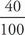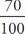# SAT Math Multiple Choice Question 125: Answer and Explanation

### Test Information

Question: 125

5. Dr. Goldberg, a noted dietician, mixes different solutions as part of her research into sugar substitutes. By weight, she mixes 40% of a sample of substitute A and 70% of a sample of substitute B to create substitute C. If Dr. Goldberg initially had 60 grams of substitute A and 110 grams of substitute B, then what would be the weight, in grams, of substitute C ?

• A. 24
• B. 77
• C. 101
• D. 170

C Dr. Goldberg takes 40% of substitute A, which consists of 60 grams. Mathematically, this can be expressed as(60) or (0.4)(60) = 24 grams. She takes 70% of substitute B, which consists of 110 grams. Mathematically, this can be expressed as(110) or (0.7)(110) = 77 grams. Substitute C will therefore consist of 24 grams + 77 grams = 101 grams, which is (C).